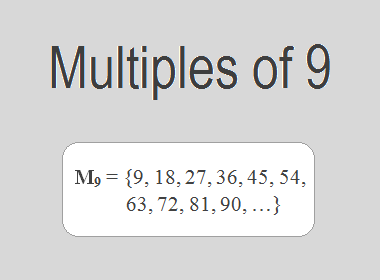# Multiples of 9The Multiples of 9 are 9, 18, 27, 36, 45, 54, 63, 72, 81, 90, … i.e. M9 = {9, 18, 27, 36, 45, 54, 63, 72, 81, 90, …}. Multiples of 9 are the series of numbers obtained by multiplying 9 with the natural numbers.

9 × 1 = 9

9 × 2 = 18

9 × 3 = 27

9 × 4 = 36

9 × 5 = 45

9 × 6 = 54

9 × 7 = 63

9 × 8 = 72

9 × 9 = 81

9 × 10 = 90

And so on.

From this series, we observe that the multiples of 9 can be derived by multiplying 9 by natural numbers in ascending order. These multiples form an arithmetic sequence with a common difference of 9.

## Properties and Patterns of Multiples of 9

Multiples of 9 possess several interesting properties and patterns that make them unique. Here are a few notable ones:

1.    Divisibility: One of the fundamental properties of multiples of 9 is their divisibility. A number is a multiple of 9 if and only if the sum of its digits is divisible by 9. For example, 36 is a multiple of 9 because 3 + 6 = 9, which is divisible by 9. This property holds true for all multiples of 9, allowing for quick divisibility tests.

2.    Cyclical Pattern: The units digit of multiples of 9 follows a cyclical pattern: 9, 8, 7, 6, 5, 4, 3, 2, 1, 0. This pattern repeats indefinitely, demonstrating the consistent nature of multiples of 9. For instance, 18, 27, 36, 45, and so on, all have a units digit of 9. This pattern can be useful in identifying the units digit of large multiples of 9.

3.    Relationship with Other Multiples: Multiples of 9 have interesting relationships with multiples of other numbers. Since 9 is a multiple of 3, any multiple of 9 is also a multiple of 3. Similarly, if we multiply 9 by any whole number, the result will be a multiple of that number. These relationships allow for exploring connections between multiples and discovering mathematical patterns.

4.    Multiplication by Powers of 10: Multiplying a multiple of 9 by powers of 10 reveals an intriguing property. When you multiply 9 by 10, the result is 90, which is a multiple of 9. This pattern extends to higher powers of 10 as well. For example, multiplying 9 by 100 gives us 900, again a multiple of 9. This property holds true for any power of 10, emphasizing the relationship between multiples of 9 and powers of 10.

********************

********************

## Applications of Multiples of 9

The study of multiples of 9 finds applications in various fields, including mathematics, science, and everyday life:

1.    LCM: One of the applications of multiples of 9 is in finding the lowest common multiple (LCM) of two or more numbers. The LCM is the lowest multiple that two or more numbers have in common. For example, to find the LCM of 9 and 6, we need to find the multiples of both numbers and identify the lowest multiple they have in common. The multiples of 9 are 9, 18, 27, 36, 45, 54, 63, 72, 81, 90, … etc. The multiples of 6 are 6, 12, 18, 24, 30, 36, 42, 48, 54, 60, … etc. The lowest multiple that they have in common is 18. Therefore, the LCM of 9 and 6 is 18.

2.    Mental Math and Calculation Tricks: Understanding the properties of multiples of 9 allows for mental calculation tricks and shortcuts. Utilizing the divisibility rule, where the sum of digits is divisible by 9, mental multiplication and division by 9 become easier and quicker. These mental math techniques can be beneficial in everyday calculations, saving time and improving number sense.

3.    Divisibility Tests: The divisibility rule based on the sum of digits of multiples of 9 is a valuable tool for performing quick divisibility tests. It finds applications in various areas, including mathematics, accounting, and finance, where divisibility plays a crucial role in determining factors, simplifying fractions, or verifying calculations.

4.    Puzzle Solving: Multiples of 9 are often used in puzzles and brain teasers. Sudoku puzzles, for example, frequently incorporate multiples of 9 in their grids, contributing to the challenge and logical reasoning required to solve them. Multiples of 9 can also be found in number sequence puzzles and riddles, stimulating critical thinking and problem-solving skills.

5.    Mathematics Education: Multiples of 9 serve as excellent teaching tools in mathematics education. They provide opportunities to explore concepts such as multiplication, division, patterns, and divisibility rules. By engaging with multiples of 9, students can strengthen their understanding of number properties, develop problem-solving strategies, and enhance their overall mathematical fluency.

6.    Mathematical Patterns and Research: Studying the patterns and properties exhibited by multiples of 9 contributes to mathematical research and exploration. Researchers analyze these patterns to uncover new insights into number theory, sequences, and algebraic structures. Multiples of 9 serve as a starting point for investigations into recurring decimals, modular arithmetic, and more.

## Conclusion:

The Multiples of 9 are the numbers obtained by multiplying 9 with natural numbers. The multiples of 9 are 9, 18, 27, 36, 45, 54, 63, 72, 81, 90, … etc. The multiples of 9 have various properties, such as divisibility, cyclical pattern, relationship with other multiples, multiplication by powers of 10, etc. The multiples of 9 have several applications in mathematics, such as finding the LCM, mental math and calculation tricks, divisibility tests, puzzle solving, mathematics education, and mathematical patterns and research.